Back-end Engineering Articles

I write and talk about backend stuff like Ruby, Ruby On Rails, Databases, Testing, Architecture / Infrastructure / System Design, Cloud, DevOps, Backgroud Jobs, and more...

@daniel_moralesp

2019-06-07

## Ruby Hashes

So far, we've seen different data types in Ruby, like Strings, Numbers, Booleans, and Arrays. Now it's the time for the following data type: Hashes.

# Arrays

A Ruby hash is a data type similar to Arrays, but instead of being zero-indexed, we can change the index value as programmers. Remember that Arrays are zero-indexed, and Ruby does that behind the scenes for us. However, we cannot change the number of that index; for instance, we cannot start the index of an array in 10; it will always start at zero. Always. So if you need for some reason to change the value of that index, or you want to have another way to identify the elements inside the data structure, you can use a Hash.

Let's see the image of the arrays again.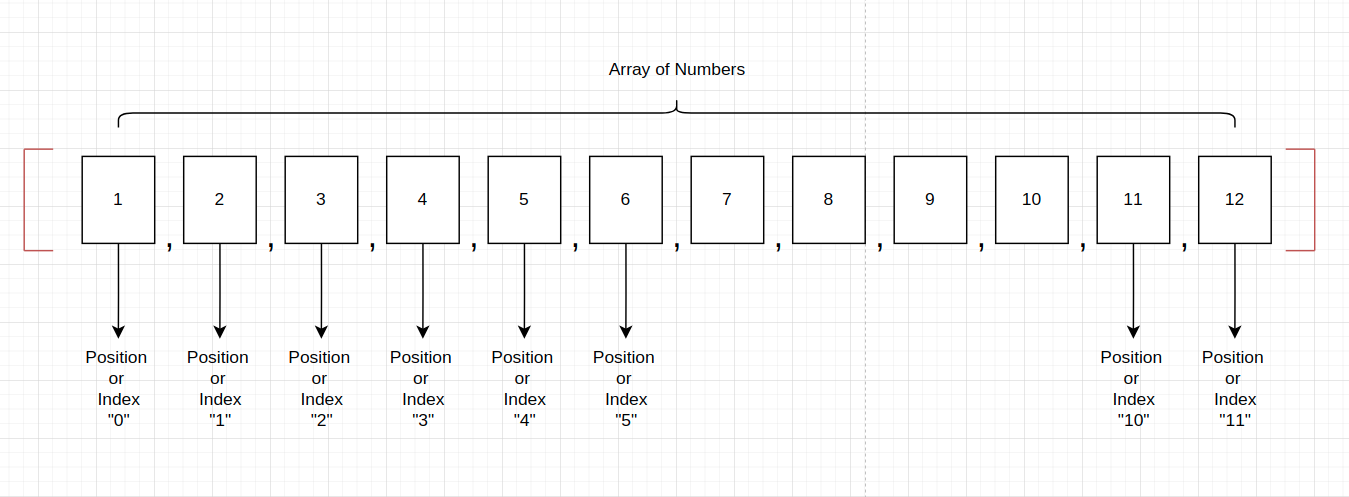With the hashes in Ruby, you can assign an index and then give it a value.

```2.6.8 :120 > array = [1, 2, 3, 4]
=> [1, 2, 3, 4] ```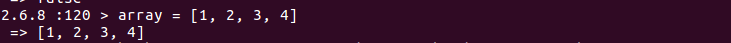# Hash

This the example of a Hash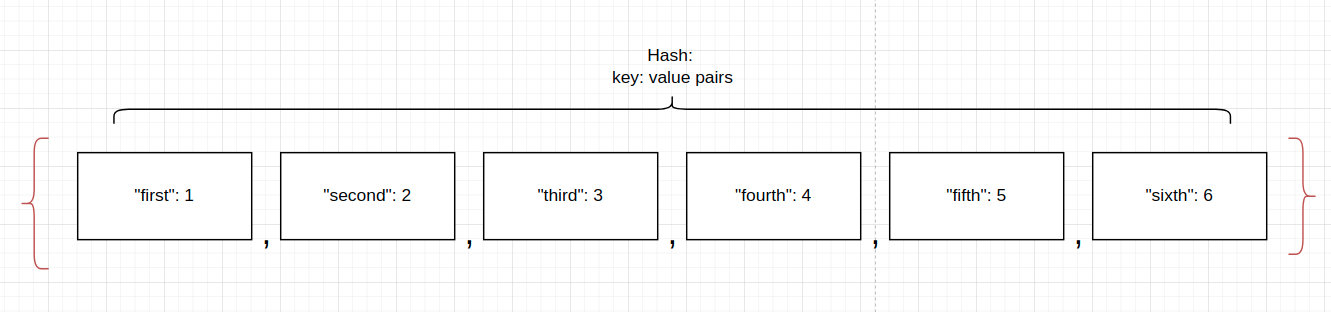What did you notice as different? First, let me explain the hash syntax and structure.

• * Now we have an open curly brace instead of a square bracket. The critical difference here in syntax
• * Then, the first element of the hash has this structure: Key:Value. The key in a hash is what we can identify as an index in a ruby array. But now, we can manipulate it and assign the value we want, a String. After the key, we have the colon punctuation ":" and after that, we have the value (similar to the elements in an array)
• * Then we have all the key:value pairs separated by a comma
• * Finally, we have the end of the curly brace.

```2.6.8 :121 > hash = {"first": 1, "second": 2, "third": 4, "fourth": 4}
=> {:first=>1, :second=>2, :third=>4, :fourth=>4} ```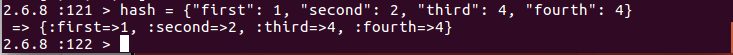Did you notice something weird in the output of the last line of code?
Look at this output: {:first=>1, :second=>2, :third=>4, :fourth=>4}

We have declared the keys as strings, but ruby returns the keys as "Symbols."

# Ruby Symbols

We currently use the syntax of the colon ":" but in previous versions of Ruby, people used many other syntaxes to separate keys with pairs. The technical name for this is hash-rocket and is denoted like so: "=>"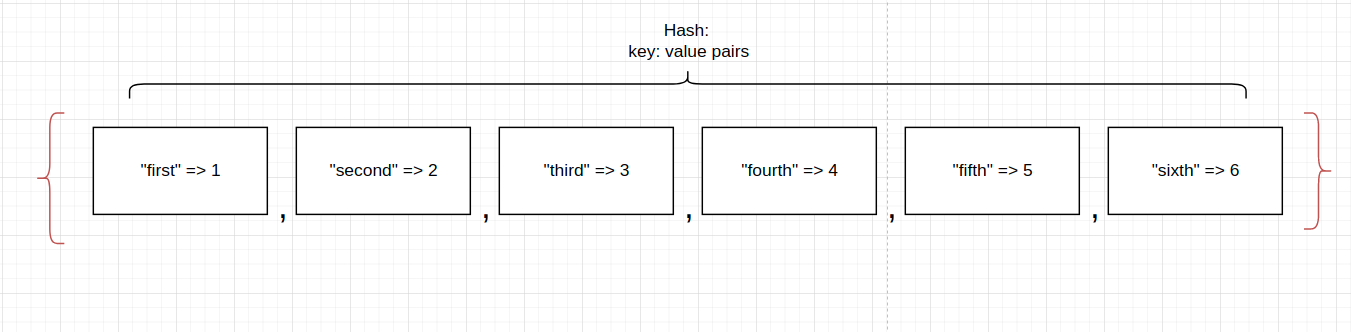But what's a symbol? A symbol looks like this:

```2.6.8 :122 > :im_a_symbol
=> :im_a_symbol
2.6.8 :123 > :hello
=> :hello
2.6.8 :124 > :one
=> :one ```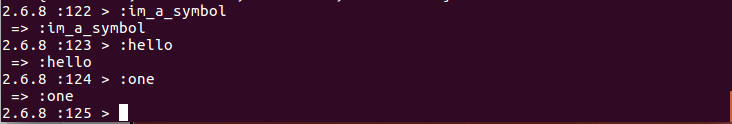We can quickly identify a symbol because it starts with a colon ":" followed by a string.

Some people confuse symbols with variables, but they have nothing to do with variables. A symbol is a lot more like a string. So what are the differences between Ruby symbols & strings? Strings are used to work with data. Symbols are identifiers. That's the main difference: Symbols are not just another kind of string; they have a different purpose.

Symbols look better; they are immutable (it cannot be modified), and if you benchmark string keys vs. symbols keys, you will find that string keys are about 1.70x slower (see more here). However, if we change the key from a string to a symbol, we must use the hash rocket notation. So we can change our first hash to be something like this.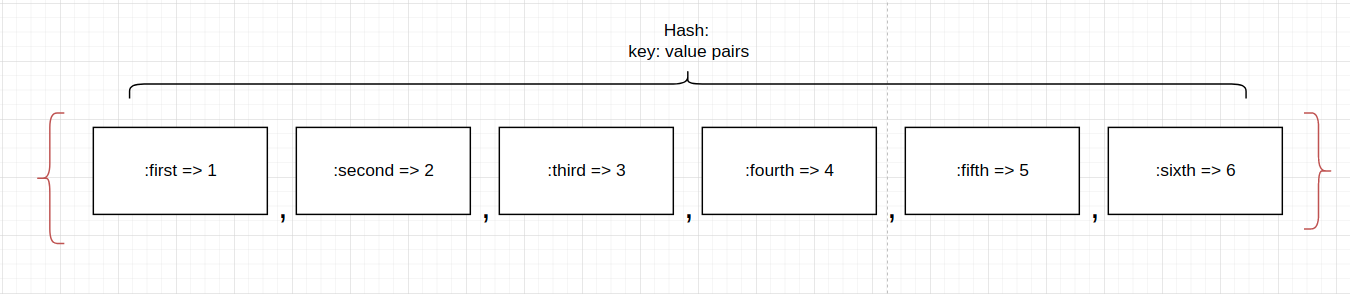```2.6.8 :125 > hash = {:first => 1, :second => 2, :third => 4, :fourth => 4}
=> {:first=>1, :second=>2, :third=>4, :fourth=>4}```So we now have the same input and output. Then given the fact that Symbols have a better performance than strings as a key inside the hashes, we'll prefer the usage of the symbols.

# Key:value pairs

The last figure shows us that the main structure of the Ruby hash is the key:value pairs. They are called pairs because they always come paired. If you forget any of them, you'll get an error. If you don't want a value, you can just put an empty string or a zero.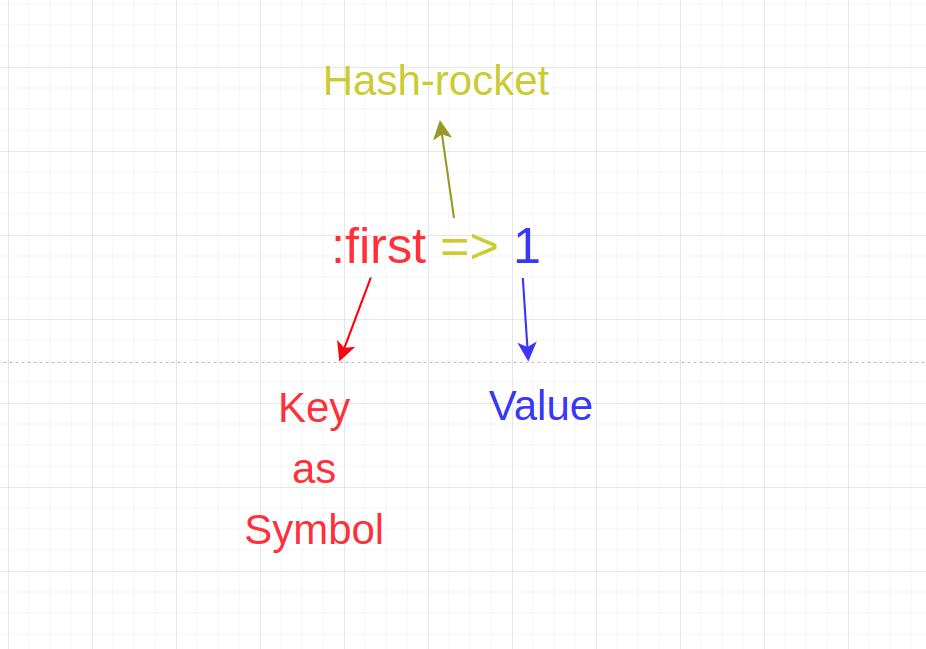# Types of Keys in a Hash

So far, we've seen keys as strings or symbols. So the question is, can I have an Integer, a Boolean, or other Ruby data types as keys in a hash?

Let's see with integers.

```2.6.8 :128 > hash = {0: 1, 1: 2, 2: 3, 3: 4}
Traceback (most recent call last):
3: from /home/daniel/.rvm/rubies/ruby-2.6.8/bin/irb:23:in `<main>'
1: from /home/daniel/.rvm/rubies/ruby-2.6.8/lib/ruby/gems/2.6.0/gems/irb-1.0.0/exe/irb:11:in `<top (required)>'
SyntaxError ((irb):128: syntax error, unexpected ':', expecting =>)
hash = {0: 1, 1: 2, 2: 3, 3: 4}```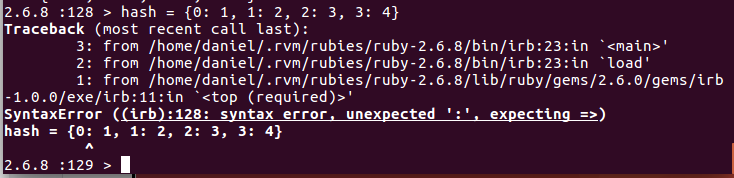If we try to create a hash using the syntax key:value and an integer as a key, we'll end up with an error. But the error says? It is a Syntax error.

SyntaxError ((irb):128: syntax error, unexpected ':', expecting =>)

Ruby is expecting a hash rocket symbol "=>" instead of a colon "." So what happens if we keep the keys but create the hash with has-rockets?

```2.6.8 :129 > hash = {0 => 1, 1 => 2, 2 => 3, 3 => 4}
=> {0=>1, 1=>2, 2=>3, 3=>4} ```

It will work! Here is the benefit of using the hash rockets because they can identify integers as keys inside the hash. This last example is how Arrays are indexed, starting from zero. The other question that can arise is if we can use integers as symbols? Let's try it.

```2.6.8 :130 > hash = {:0 => 1, :1 => 2, :2 => 3, :3 => 4}
Traceback (most recent call last):
3: from /home/daniel/.rvm/rubies/ruby-2.6.8/bin/irb:23:in `<main>'
1: from /home/daniel/.rvm/rubies/ruby-2.6.8/lib/ruby/gems/2.6.0/gems/irb-1.0.0/exe/irb:11:in `<top (required)>'
SyntaxError ((irb):130: syntax error, unexpected tINTEGER, expecting tSTRING_CONTENT or tSTRING_DBEG or tSTRING_DVAR or tSTRING_END)
hash = {:0 => 1, :1 => 2, :2 => 3, :3 =...
^```So the answer is in error itself. Ruby is expecting a string as a symbol. Remember that symbols are similar to strings, but they help us identify in hashes.

Another question, can we have Booleans as keys? Yes, we can; let's do it.

```2.6.8 :131 > hash = {true => 1, false => 2}
=> {true=>1, false=>2} ```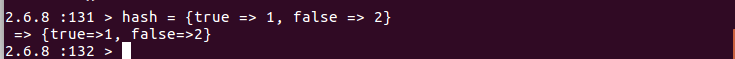But what will happen if we repeat a key with the same name?

```2.6.8 :132 > hash = {true => 1, false => 2, false => 3}
=> {true=>1, false=>3} ```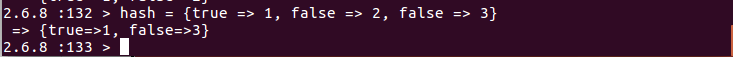It will take just the last declared element; the same thing happens with symbols (even we'll receive a warning)

```2.6.8 :133 > hash = {:first => 1, :second => 2, :second => 3}
(irb):133: warning: key :second is duplicated and overwritten on line 133
=> {:first=>1, :second=>3} ```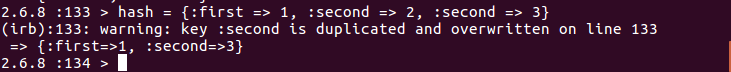Can you see the beauty of all of this?

# Accessing hash values

Now we probably want to access the values of our hash. First, we can call the key, and then we'll see the value printed out.

Note: please take care about what we'll do here because it is a bit complex and mix the knowledge we already have about hashes

Let's digest line by line.

hash_one

```2.6.8 :152 > hash_one = {:first => 1, :second => 2, :third => 3, :fourth => 4}
=> {:first=>1, :second=>2, :third=>3, :fourth=>4}
2.6.8 :153 > hash_one[:first]
=> 1
2.6.8 :154 > hash_one[:second]
=> 2 ```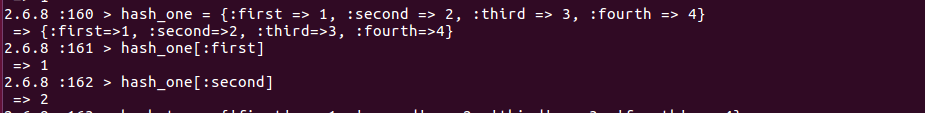Here we've declared a hash with symbols and hash rockets. So when we want to access a value, we have to call it similar to an array, but instead of passing the position/index of the array, we've to give the key of the hash we want to retrieve

hash_two

```2.6.8 :163 > hash_two = {'first' => 1, 'second' => 2, 'third' => 3, 'fourth' => 4}
=> {"first"=>1, "second"=>2, "third"=>3, "fourth"=>4}
2.6.8 :164 > hash_two[:first]
=> nil
2.6.8 :165 > hash_two['first']
=> 1 ```Now we've declared a hash with strings as keys and with hash rockets. So we've to take care of the way we call any value because if we try to call it like a symbol hash_two[:first], we'll have nil as a result. So we have to call it as a string: hash_two['first']

hash_three

```2.6.8 :166 > hash_three = {'first': 1, 'second': 2, 'third': 3, 'fourth': 4}
=> {:first=>1, :second=>2, :third=>3, :fourth=>4}
2.6.8 :167 > hash_three['first']
=> nil
2.6.8 :168 > hash_three[:first]
=> 1 ```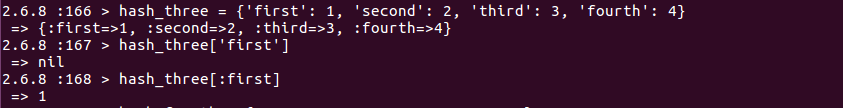In hash_three we've created a hash with strings as keys and colons ":" instead of hash rockets. But if you see this line of code hash_three['first'] returns nil, but if we call the value with the symbol syntax, we'll get the correct value hash_three[:first], which is something weird, is a behavior we need to take care because we can end up with nil values when we try to return something from a hash declared on this way

hash_fourth

```2.6.8 :169 > hash_fourth = {0 => 1, 1 => 2, 2 => 3, 3 => 4}
=> {0=>1, 1=>2, 2=>3, 3=>4}
2.6.8 :170 > hash_fourth
=> 1
2.6.8 :171 > hash_fourth['0']
=> nil
2.6.8 :172 > hash_fourth[:0]
Traceback (most recent call last):
3: from /home/daniel/.rvm/rubies/ruby-2.6.8/bin/irb:23:in `<main>'
1: from /home/daniel/.rvm/rubies/ruby-2.6.8/lib/ruby/gems/2.6.0/gems/irb-1.0.0/exe/irb:11:in `<top (required)>'
SyntaxError ((irb):172: syntax error, unexpected tINTEGER, expecting tSTRING_CONTENT or tSTRING_DBEG or tSTRING_DVAR or tSTRING_END)
hash_fourth[:0]
^
2.6.8 :173 > ```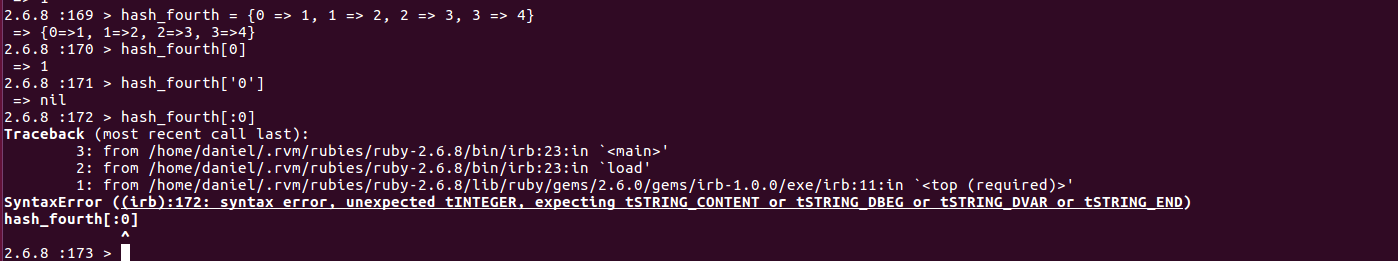Finally, we have the hash, which contains integers as keys and is declared with hash rockets. We can retrieve the information as an array, just passing the integer key we want to return hash_fourth, but if we try to return it as a string, we get a nil value hash_fourth['0'], and if we try to retrieve it as a symbol, we get an error. So that's another weird behavior we have to take care of.

# Adding an element to a hash

As with Arrays, we can start empty hashes and add elements to them. The ways we can do these two things are straightforward.

```2.6.8 :180 > new_hash = {}
=> {}
2.6.8 :181 > new_hash[:first] = 1
=> 1
2.6.8 :182 > new_hash['second'] = 2
=> 2
2.6.8 :183 > new_hash
=> {:first=>1, "second"=>2}```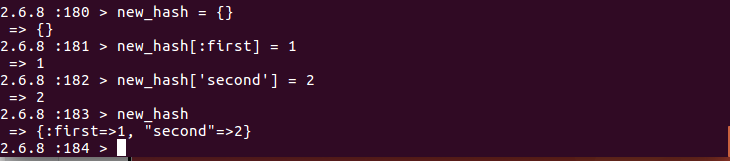Let's explain:

• * First line of code creates an empty hash with the syntax realist to Ruby hashes: "{}"
• * Then, we started adding new elements. The first element added, we did it with the symbol syntax, given the key, and then assigning the value: new_hash[:first] = 1
• * In the last line, we did the same thing, but we used "string" as a key for the hash. new_hash['second'] = 2
• * Finally, we printed out the final hash

# Modifying hash values

Once we have a hash, we can modify the internal values like so

```2.6.8 :186 > new_hash = {:first=>1, "second"=>2}
=> {:first=>1, "second"=>2}
2.6.8 :187 > new_hash['second'] = 3
=> 3
2.6.8 :188 > new_hash
=> {:first=>1, "second"=>3}```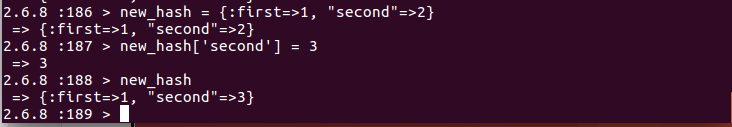We've created a new_hash, and then we get the key 'second' like this: new_hash['second'], and after that, we assign a new value, in this case, the Integer 3. When we printed it out, we saw the new value assigned to this key

# Deleting hash elements

We can also delete hash elements, in this case using an especial method from Ruby called ".delete"

```2.6.8 :189 > new_hash = {:first=>1, "second"=>2}
=> {:first=>1, "second"=>2}
2.6.8 :190 > new_hash.delete(:first)
=> 1
2.6.8 :191 > new_hash
=> {"second"=>2} ```The ".delete" method receives the key we want to delete, in this case (:first). And then, when we print the result, we've just one element now.

# Iterating over a hash

As with Ruby arrays, we can iterate over hashes. But this time, we have to consider each key and value pair and use the "each" method we saw in a previous post.

```2.6.8 :201 > hash = {'first': 1, 'second': 2, 'third': 3, 'fourth': 4}
=> {:first=>1, :second=>2, :third=>3, :fourth=>4}
2.6.8 :202 > hash.each do |key, value|
2.6.8 :203 >     puts key, value
2.6.8 :204?>   end
first
1
second
2
third
3
fourth
4
=> {:first=>1, :second=>2, :third=>3, :fourth=>4} ```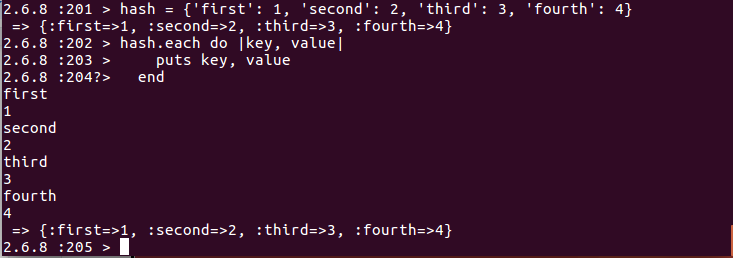Let's explain these lines of code:

• * First, we call the variable which has the hash assigned
• * Next to it, we run the method ".each" to iterate over it
• * After that, we open the Ruby block with the keyword "do."
• * At the end of the first line, we now have two variables inside the pipe symbol "|key, value|". The names don't matter; what matters is the position of each one because the first will permanently save the key and the second the value. It's pretty similar to the array iteration; the only difference is now we have 2 values that we can manipulate (keys and values)
• * Inside the body of the ruby block, we have a "puts" keyword to print both of them: key and value
• * Finally, we close the ruby block with the keyword "end"

Exciting, isn't it?

# Other Ruby hash methods

This is the last thing we'll be doing with hashes. We have a list of built-in methods to do everyday tasks with hashes. We have a lot, so if you want to know the exhaustive list, here you can go and see all the methods in the left panel. https://ruby-doc.org/core-2.5.1/Hash.html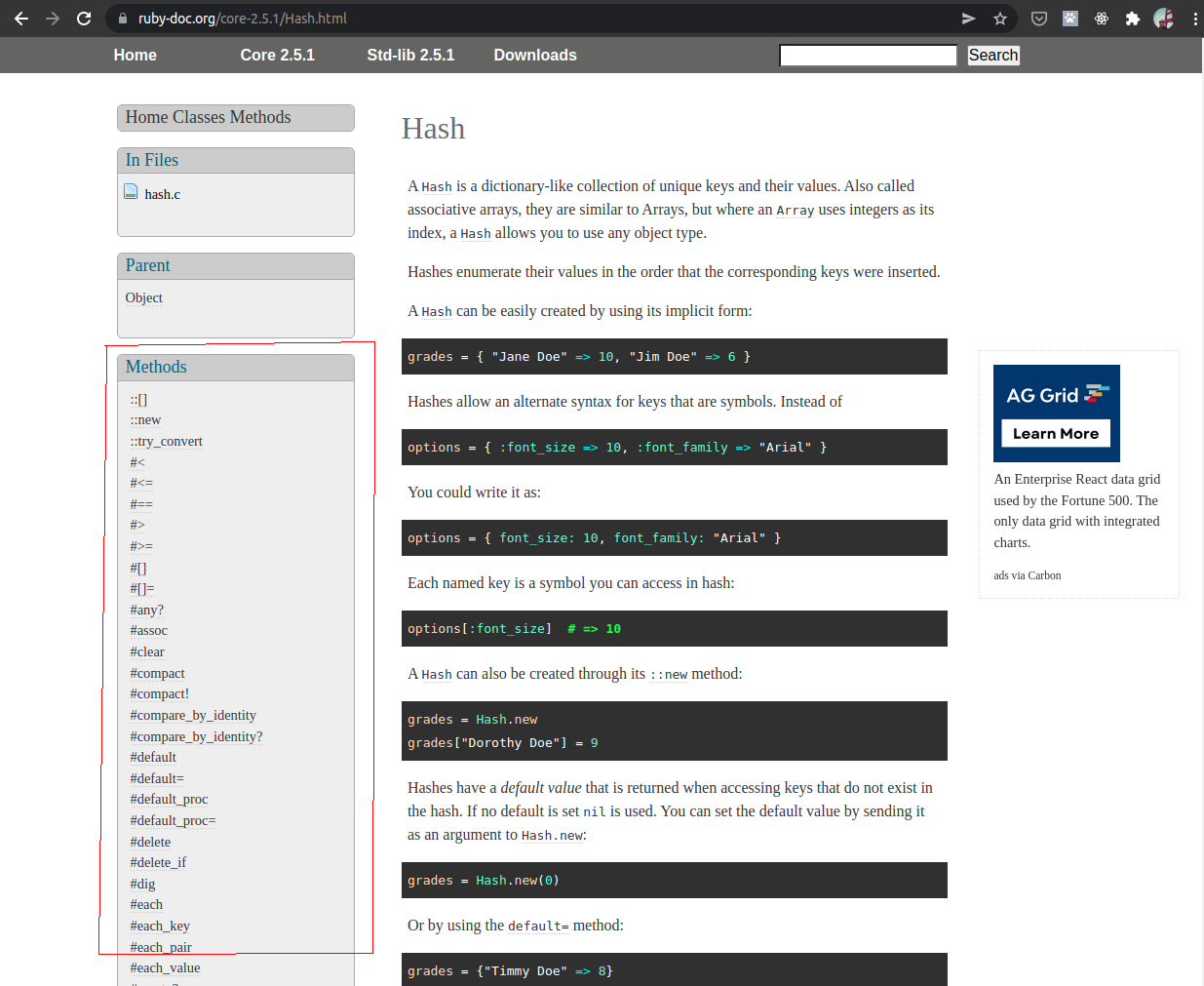But let's see some important ones.

```2.6.8 :215 > hash = {'first': 1, 'second': 2, 'third': 3, 'fourth': 4}
=> {:first=>1, :second=>2, :third=>3, :fourth=>4}
2.6.8 :216 > hash.length
=> 4
2.6.8 :217 > hash.has_key?(:first)
=> true
2.6.8 :218 > hash.has_key?(:tenth)
=> false
2.6.8 :219 > hash.keys
=> [:first, :second, :third, :fourth]
2.6.8 :220 > hash.values
=> [1, 2, 3, 4] ```

Let's check line by line:

• * First, we've created the hash
• * Then we asked for the length of key:paris and we got 4
• * Then we asked if the hash has a key with the symbol ":first" and the result was true
• * Then we asked if the hash has a key with the symbol ":tenth" and the result was false
• * Then we asked for the keys, and we got the key names
• * Finally, we've asked for the values, and we got the value names

Pretty awesome!

Hope you learned a lot about hashes